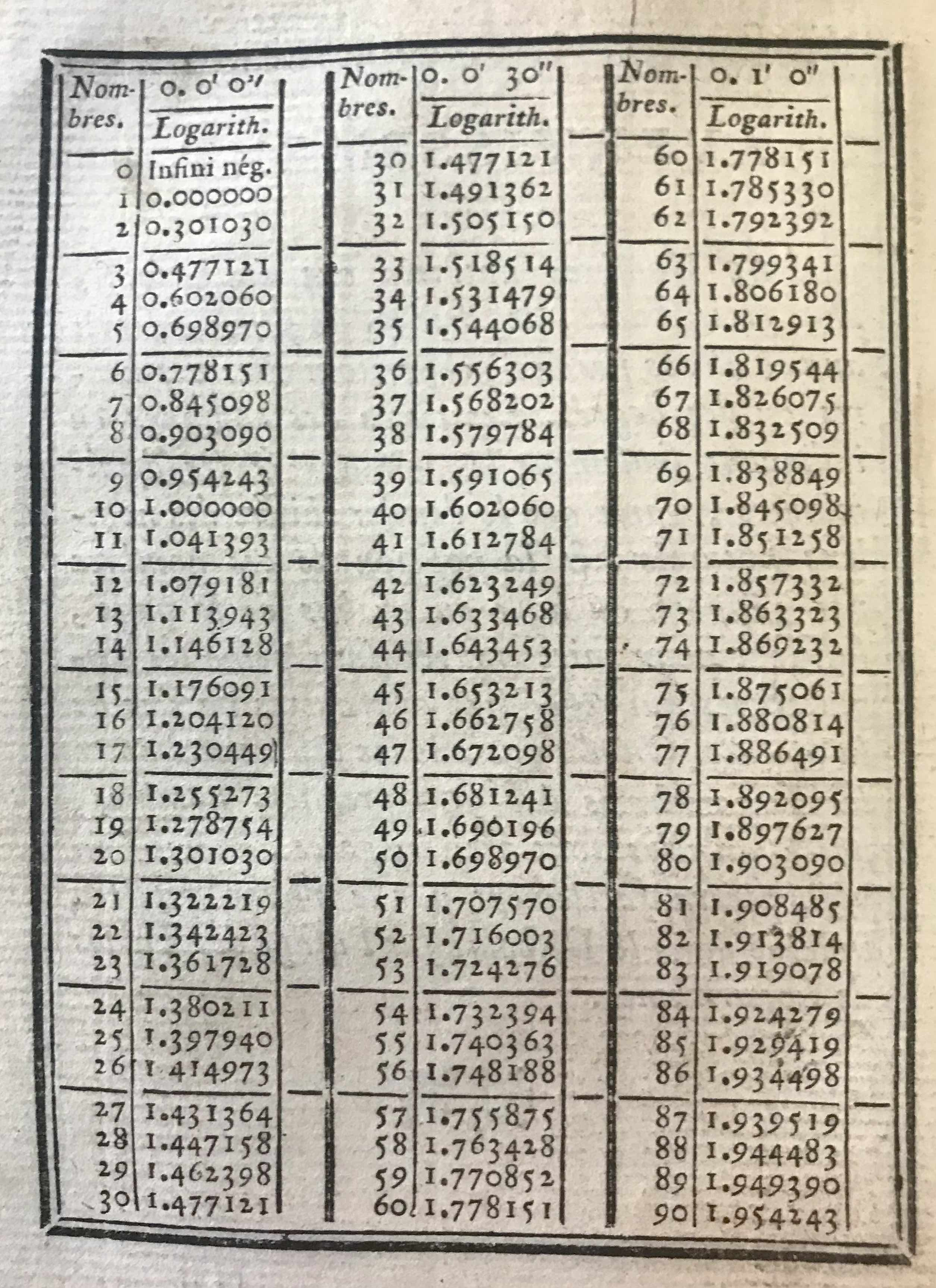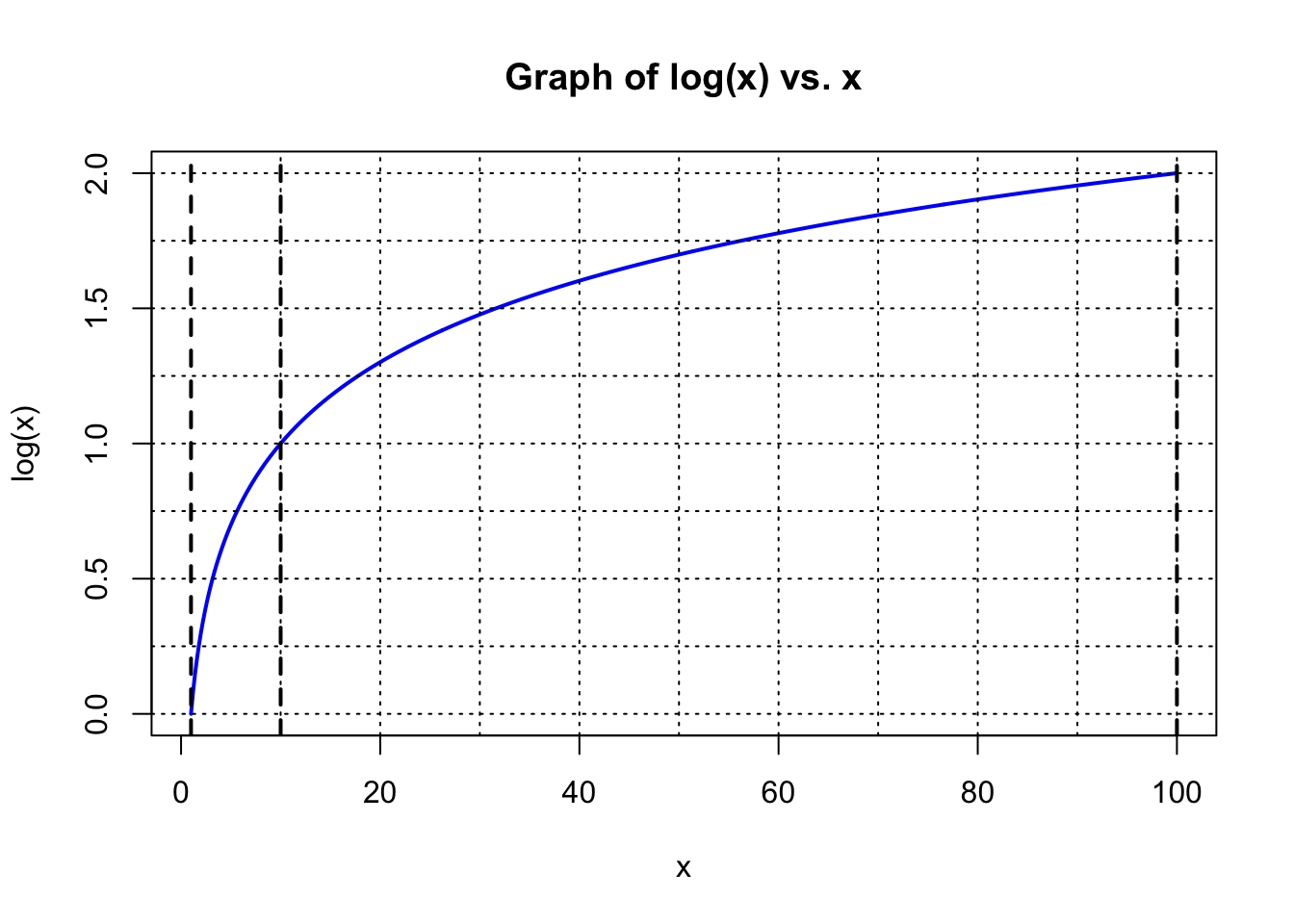## 1.2 Logarithms and Log Scales

Consider an arbitrary positive real number $$x$$. As we just discussed, we can express $$x$$ in terms of a base number – say, $$b$$ – raised to a power $$p$$:

$x = b^p.$ In standard mathematical language we say that the logarithm of the number $$x$$ is the exponent $$p$$: $\log_b x = p.$ The above expression is read, “The log, base $$b$$, of $$x$$ is equal to $$p$$.” This definition leads to the following identity:

$x = b^{\log_b x}.$

In mathematical terms we say that the function $$\log_b x$$ is the inverse of the function $$b^x$$ – if we start with $$x$$ and find $$b^x$$, and then take the logarithm using base $$b$$ of the resulting number, we get $$x$$ back again: $\log_b b^x = x.$

In our earlier example we used $$2$$ as a base, but the most common base to use, which is what typical slide rules have been based upon (no pun intended), is $$b$$ = 10, or “Base 10”: $$x = 10^p$$. The standard is simply to define the logarithm – or, the common logarithm – as that using Base 10:

${\rm if} ~~ x = 10^p ~~~~ \longrightarrow ~~~~ \log x ~ \equiv ~ \log_{10} x = p.$

In other words, ask, “To what power must you raise the number 10 in order to obtain the number $$x$$?” The answer, $$p,$$ is the logarithm of $$x$$ (simply called “log of $$x$$”). The convention is to write $$\log x"$$ when the base being used is 10. If it were another base, say $$2$$, then the expression would be written $$\log_2 x$$.

We saw earlier that any number raised to the power of “zero” is by definition “one.” Hence, log 1 = 0. That is, 1 = $$10^0$$. The logarithm of a number greater than 1 will be a positive number. For example, $$10 = 10^1$$ and $$100 = 10^2$$, so $$\log 10 = 1$$ and $$\log 100 = 2$$. The logarithm of a number less than 1 will be negative: $$0.1 = 10^{-1}$$ and $$0.01 = 10^{-2}$$, hence $$\log 0.1 = -1$$ and $$\log 0.01 = -2$$. What about other, more general numbers? As we did before for our “Base 2” example, we can take the square root of 10, which is $$10^{1/2}$$ = $$10^{0.5}$$ = $$\sqrt{10}$$ = 3.1623, and hence we can infer that $$\log 3.1623 = 0.5$$. It can also be verified that $$10^{0.30103}$$ yields 2.0000. Thus, $$\log 2$$ is approximately equal to 0.30103. Precise values of the common logarithms of numbers can be calculated by using calculus, finite difference techniques, or other methods, and their values have been computed and tabulated with various degrees of accuracy over the past centuries. One such method for computing logarithms is presented in the next chapter. Books containing tables of values of logarithms were painstakingly produced and published for use in the types of calculations that will be described below; these books are also collectible and can be found in many used book stores.A table of logarithms from the year 1781.2

Modern electronic computer languages have built-in algorithms that can produce logarithms of numbers to high accuracy. The following is a table of the Base 10 common logarithms of integer numbers between 1 and 100, similar to what can be found in a book of logarithms, but here generated by a modern personal computer:

x log(x) x log(x) x log(x) x log(x)
1 0.00000 26 1.41497 51 1.70757 76 1.88081
2 0.30103 27 1.43136 52 1.71600 77 1.88649
3 0.47712 28 1.44716 53 1.72428 78 1.89209
4 0.60206 29 1.46240 54 1.73239 79 1.89763
5 0.69897 30 1.47712 55 1.74036 80 1.90309
6 0.77815 31 1.49136 56 1.74819 81 1.90849
7 0.84510 32 1.50515 57 1.75587 82 1.91381
8 0.90309 33 1.51851 58 1.76343 83 1.91908
9 0.95424 34 1.53148 59 1.77085 84 1.92428
10 1.00000 35 1.54407 60 1.77815 85 1.92942
11 1.04139 36 1.55630 61 1.78533 86 1.93450
12 1.07918 37 1.56820 62 1.79239 87 1.93952
13 1.11394 38 1.57978 63 1.79934 88 1.94448
14 1.14613 39 1.59106 64 1.80618 89 1.94939
15 1.17609 40 1.60206 65 1.81291 90 1.95424
16 1.20412 41 1.61278 66 1.81954 91 1.95904
17 1.23045 42 1.62325 67 1.82607 92 1.96379
18 1.25527 43 1.63347 68 1.83251 93 1.96848
19 1.27875 44 1.64345 69 1.83885 94 1.97313
20 1.30103 45 1.65321 70 1.84510 95 1.97772
21 1.32222 46 1.66276 71 1.85126 96 1.98227
22 1.34242 47 1.67210 72 1.85733 97 1.98677
23 1.36173 48 1.68124 73 1.86332 98 1.99123
24 1.38021 49 1.69020 74 1.86923 99 1.99564
25 1.39794 50 1.69897 75 1.87506 100 2.00000

Table of Common Logarithms

Taking a few examples, the values in the table tell us that the number 7 is equivalent to $$10^{0.8451}$$, 12 = $$10^{1.07918}$$, 63 = $$10^{1.79934}$$, and 100 = $$10^2$$. In the following curve the value $$x$$ is read on the horizontal axis and its logarithm is read on the vertical axis:The heavy vertical dashed lines in the plot above are at values of $$x$$ = $$10^0$$, $$10^1$$, and $$10^2$$, and we see that they cross the blue curve at values of 0, 1, and 2 on the left-hand scale. Now suppose that we stretch the numerical scaling of the horizontal axis to create a linear relationship to the values of logarithms along the vertical axis. Here is the result:The special scaling along the $$x$$ axis shown in the final figure above is that found on the standard scales of a slide rule. The figure shows that with this particular scaling on the slide rule, the distance from the number 1 to the number $$x$$ is directly proportional to the logarithm of the number $$x$$. Notice that the distance from 1 to 10 along the horizontal axis is the same as the distance from 10 to 100 (as would be the distance between 100 and 1000 or between 0.1 and 1, and so forth). Every factor of 10 changes the logarithm by one unit. One can see also that the logarithm of 20 (distance from 1 to 20) is the same as the logarithm of 10 (distance from 1 to 10) plus the logarithm of 2 (distance from 1 to 2).

1. Tables de Logarithmes, Chez veuve DESAINTE, Libraire, rue du Foin, Paris, 1781.↩︎